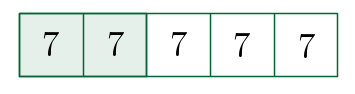# Teaching Strategy

MathConcept uses a set of math tricks to help kids to learn basic math. The highlights are:

## Mental Technique

The method of using combination of 10 helps students to add or subtract numbers without using their fingers. In the mean time, it encourages students to do math in their head more often.

$$8+7$$

Instead of working through the problem traditionally by hand using vertical form, students are taught to think mentally as follow:

\begin{align*}8+7 &= 8+2+5 \\ &= 10+5 \\ &= 15 \end{align*}

Combination of 10 can also be applied on subtraction.

For example, in subtraction

$$24-18$$

Instead of using borrowing method in vertical form, students could do the following:

\begin{align*}24-18 &= 24-14-4 \\ &= 10-4 \\ &= 6 \end{align*}

## Simple Language

We ensure students are being taught in simple language that every kid can understand, by breaking down the meaning of root words in the mathematical context. Students are also trained to write down the steps logically and clearly.

## Learned by Drawing

Significant portion of MathConcept printed courseware contain meaningful pictures, charts, and tables to help explain different concepts. Offering students deeper insights into problems that transcend the written words.

For example,

$$\text{finding }\frac{2}{5}\text{ of }35$$

Student can first draw 5 boxes and fill in each box with the number 7 because in multiplication table, 5 times 7 is 35.And now add the numbers in the colored boxes gives us

$$7+7 = 14$$

## Using Concrete Example

Teaching tools are used to help introducing, explaining, and reinforcing concepts and skills. By actually doing the math themselves helps students to learn to analysis the problem, organize the logical steps, and present the final solution to the teacher.

## Word Problems Practice

MathConcept provides plentiful written practices with computation for the the systematic development of mathematical thought and skills. Our printed courseware covers the entire spectrum from practising number writing to more advanced mathematics such as calculus.